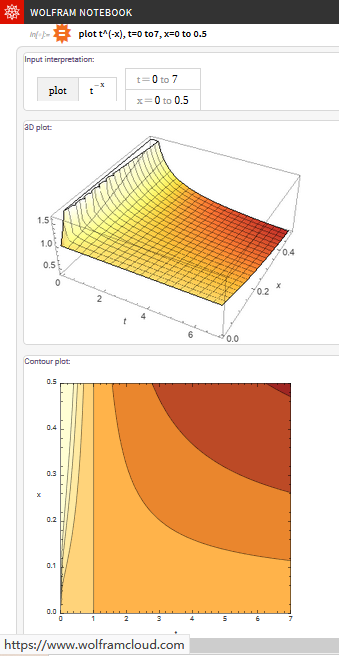#### Blog Stats

• 117,407 hits

# The Asymptotic Convergence-Rate of Q-learning

### The Asymptotic Convergence-Rate of Q-learning

the-asymptotic-convergence-rate-of-q-learning

The asymptotic rate of convergence of Q-learning is Ο( 1/tR(1-γ) ), if R(1-γ)<0.5, where R=Pmin/Pmax, P is state-action occupation frequency.

|Qt (x,a) − Q*(x,a)| < B/tR(1-γ)

Convergence-rate is the difference between True value and Optimum value, i.e., the smaller it is, the faster convergence Q-learning is. We hope the Ο( 1/tR(1-γ) ) should be as small as possible, which means the R is bigger, i.e., the on-policy distribution is higher, the state space should be smaller.

https://www.wolframcloud.com/objects/zp21300/Published/The_Asymptotic_Convergence-Rate_of_Q-learning.nbSidebar